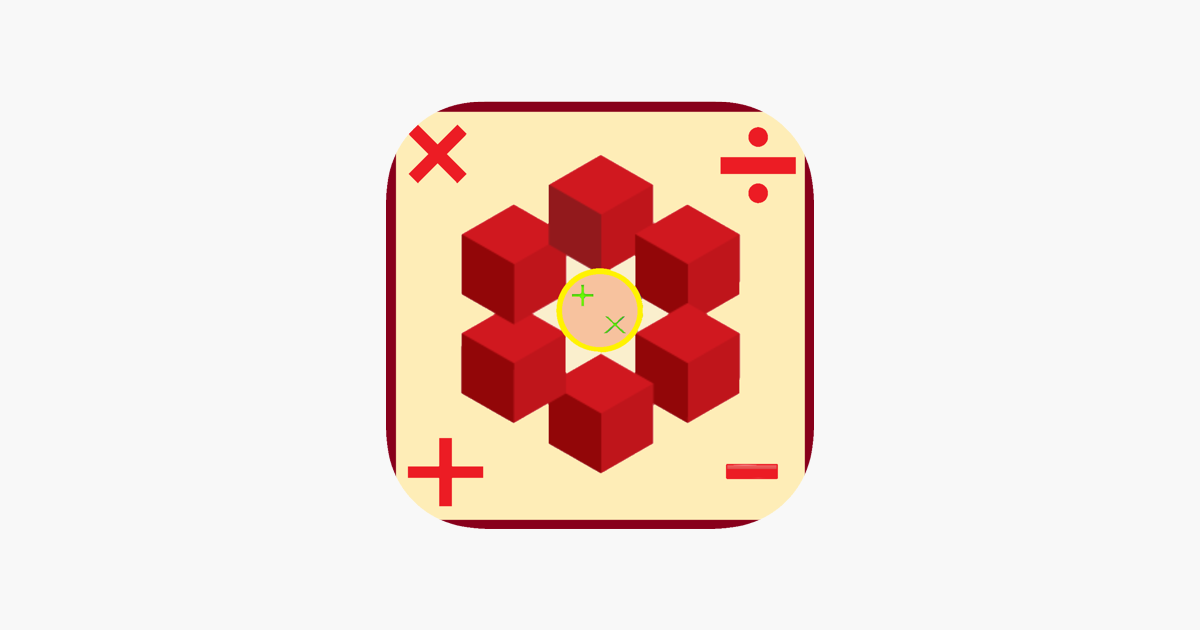# Maths Lessons – Alva JacobsenA simple app to learn basics of maths including mathematical operations e.g. addition, subtraction, multiplication , division etc. symbols, name of different geometric symbols e.g. straight, intersection, union etc , counting in words and numbers from 0 to 100 and some knowledge of important & basic numbers used in daily life .

1)Maths Symbol – In this part of app ,user are shown images and names of several mathematical operations like addition, subtraction, multiplication, division ,equality ,inequality etc.Pronunciation is also added to help user to learn the correct pronunciation of every symbol.

2)Geometric Lines Name – In this part of app ,user are shown images and names of several Geometric Lines Name like straight, diagonal, vertical, parallel ,curved ,dotted etc.Pronunciation is also added to help user to learn the correct pronunciation of every Geometric Lines Name.

3)Hundred Chart – In this part of app ,user are shown numbers from 0 to 100 in digits and words like 0, zero etc.Pronunciation is also added to help user to learn the correct pronunciation of every number from 0 to 100.

4)Number Chart – In this part of app, user can learn how some special and different units are read like 32% read as Thirty Two Percent,8.3 read as Eight point three,\$2.66 read as Two dollars sixty six cents etc.Pronunciation is also added to help user to learn the correct pronunciation & for better understanding.

5) Test Yourself – In this part of app ,user will be asked a question and for each question they will be provided four images as options.User have to select the answer out of those four options.If the answer chosen will be correct the answer will be marked as correct and if the answer chosen will be wrong it will be marked as incorrect.